# KR20160010120A - Stereo matching apparatus and method using unary confidences learning and pairwise confidences learning - Google Patents

Stereo matching apparatus and method using unary confidences learning and pairwise confidences learning Download PDF

## Info

Publication number
KR20160010120A
KR20160010120A KR1020140091042A KR20140091042A KR20160010120A KR 20160010120 A KR20160010120 A KR 20160010120A KR 1020140091042 A KR1020140091042 A KR 1020140091042A KR 20140091042 A KR20140091042 A KR 20140091042A KR 20160010120 A KR20160010120 A KR 20160010120A
Authority
KR
South Korea
Prior art keywords
reliability
confidence measure
current pixel
learning
cost
Prior art date
Application number
KR1020140091042A
Other languages
Korean (ko)
Inventor
최욱
장현성
Original Assignee
삼성전자주식회사
Priority date (The priority date is an assumption and is not a legal conclusion. Google has not performed a legal analysis and makes no representation as to the accuracy of the date listed.)
Filing date
Publication date
Application filed by 삼성전자주식회사 filed Critical 삼성전자주식회사
Priority to KR1020140091042A priority Critical patent/KR20160010120A/en
Publication of KR20160010120A publication Critical patent/KR20160010120A/en

• 230000013016 learning Effects 0 title 2

## Images

•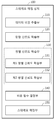•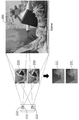•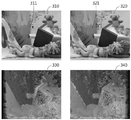•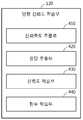•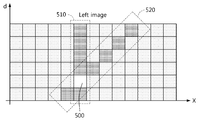•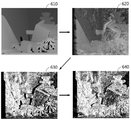•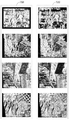•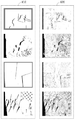•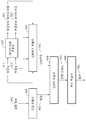•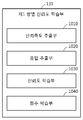•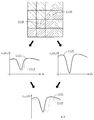•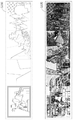•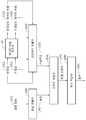•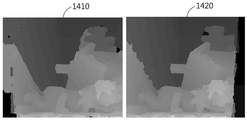•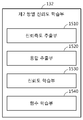•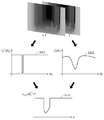•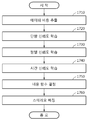•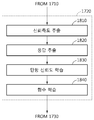••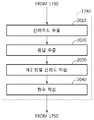## Classifications

• GPHYSICS
• G06COMPUTING; CALCULATING; COUNTING
• G06TIMAGE DATA PROCESSING OR GENERATION, IN GENERAL
• G06T7/00Image analysis
• G06T7/50Depth or shape recovery
• G06T7/55Depth or shape recovery from multiple images
• G06T7/593Depth or shape recovery from multiple images from stereo images
• HELECTRICITY
• H04ELECTRIC COMMUNICATION TECHNIQUE
• H04NPICTORIAL COMMUNICATION, e.g. TELEVISION
• H04N13/00Stereoscopic video systems; Multi-view video systems; Details thereof
• H04N2013/0074Stereoscopic image analysis
• H04N2013/0081Depth or disparity estimation from stereoscopic image signals

## Abstract

Disclosed are an apparatus and a method of stereo matching using unary confidence learning and pairwise confidence learning. The stereo matching method comprises the following steps: learning pairwise confidence which represents a relationship between a current pixel and a neighboring pixel; determining a cost function of stereo matching based on the pairwise confidence; and stereo matching a left image and a right image with the minimum costs using the cost function.

## Description

 STEREO MATCHING APPARATUS AND METHOD USING UNARY CONFIDENCES LEARNING AND PAIRWISE CONFIDENCES LEARNING 

One embodiment of the present invention relates to a stereo matching apparatus and method, and more particularly, to an apparatus and method for stereo matching at a minimum cost using a cost function determined by learning reliability associated with a pixel.

As 3D image reproduction technology using left and right images is commercialized, stereo matching for finding corresponding pixels between a left image and a right image has been developed.

Stereo matching can output the binocular parallax information of the left image and the parallax information of the right image by matching the left image and the right image. Specifically, the stereo matching can determine the data cost for determining the similarity between the pixels of the right image and the pixels of the left image, which may correspond to the pixels of the left image. Next, the stereo matching can determine the binocular disparity information that minimizes the cost function including the data cost as the binocular disparity information of the left image and the disparity information of the right image.

However, since the size of an object included in a left image or a right image is larger than a pixel, and pixels included in each of the objects are similar in color, a pixel of the right image, which does not correspond to the pixel of the left image, The binocular parallax may be smaller than the binocular disparity between the pixels of the right image corresponding to the left image and the pixels of the left image. In this case, an error occurs in minimizing the cost function, so that the incorrect binocular disparity information can be determined as the binocular disparity information of the left image and the binocular disparity information of the right image.

Therefore, a cost function that minimizes errors in the stereo matching process is required.

A stereo matching method according to an exemplary embodiment of the present invention includes learning pairwise confidence indicating a relationship between a current pixel and neighboring pixels; Determining a cost function of stereo matching based on the first pairwise reliability; And stereo matching the left and right images at a minimum cost using the cost function.

Learning the pairwise reliability of the stereo matching method according to an embodiment includes learning a first pair reliability indicating a relation between a current pixel and a neighboring pixel included in a current frame; Or learning a relation between a current pixel included in the current frame and a neighboring pixel included in the previous frame.

The step of learning the first pair reliability of the stereo matching method includes calculating a similarity between the binocular disparity of the current pixel and the binocular disparity of the neighboring pixels from the data cost of the left image, Extracting an associated confidence measure; Extracting a response to the confidence measure from the verified discontinuity information; And learning the relationship between the extracted confidence measure and the response.

The step of extracting the confidence measure of the stereo matching method according to an embodiment may comprise the steps of: obtaining a confidence measure for color similarity between the current pixel and the neighboring pixel, a confidence measure for the position similarity between the current pixel and the neighboring pixel, And a confidence measure for the binocular disparity similarity between the neighboring pixels.

The step of learning the second pair reliability of the stereo matching method according to an exemplary embodiment may include calculating the similarity between the binocular disparity of the previous frame and the binocular disparity of the current frame from the data cost of the left image, Extracting an associated confidence measure; Extracting a response to the confidence measure from the verified binarized video (Disparity video); And learning the relationship between the extracted confidence measure and the response.

The step of determining the cost function of the stereo matching method according to an exemplary embodiment may further include using the data cost of the left image, the data cost of the right image, the similarity between the binocular disparity of the current pixel and the binocular disparity of the neighboring pixels, To determine the cost function.

The method of stereo matching according to an embodiment further comprises learning unary confidence associated with the data cost of the current pixel, and the step of determining the cost function is based on the unary reliability and the binaural reliability, The cost function of the matching can be determined.

The step of learning the unary reliability of the stereo matching method according to an exemplary embodiment of the present invention includes calculating a reliability of a current pixel based on whether a current pixel is included in an occlusion area from a data cost of a left image, Extracting a measure; Extracting a response to the trust measure from the validated coverage area information; And learning the relationship between the extracted confidence measure and the response.

The step of extracting the confidence measure of the stereo matching method according to an embodiment may comprise the steps of determining the uniqueness of the minimized data cost, the confidence measure for the specificity, the confidence measure as to whether the current pixel is included in the occlusion region, And at least one of the confidence measures for the user.

Learning the unidirectional reliability of the stereo matching method includes extracting a confidence measure related to the accuracy of the binocular disparity of the current pixel from the data cost of the left image, the right image, the left image, and the data cost of the right image; Extracting a response to the confidence measure from the verified binocular disparity information; And learning the relationship between the extracted confidence measure and the response.

The step of extracting the confidence measure of the stereo matching method according to an exemplary embodiment of the present invention includes a confidence measure as to whether or not the data cost of the minimized left image is equal to the data cost of the right image with the minimized data, A confidence measure, a confidence measure, and a confidence measure for a texture contained in the current pixel.

A stereo matching method according to an exemplary embodiment of the present invention includes learning unary confidence indicating whether a current pixel is included in an occlusion area; Determining a cost function of stereo matching based on the unary reliability; And stereo matching the left and right images at a minimum cost using the cost function.

The step of learning the unary reliability of the stereo matching method according to an exemplary embodiment of the present invention includes calculating a reliability of a current pixel based on whether a current pixel is included in an occlusion area from a data cost of a left image, Extracting a measure; Extracting a response to the trust measure from the validated coverage area information; And learning the relationship between the extracted confidence measure and the response.

The stereo matching method according to an exemplary embodiment further includes learning pairwise confidence indicating a relationship between a current pixel and neighboring pixels, and the step of determining the cost function is based on the unary reliability and the pair reliability The cost function of the stereo matching can be determined.

A stereo matching apparatus according to an exemplary embodiment of the present invention includes: a pair reliability learning unit that learns pairwise confidence indicating a relationship between a current pixel and neighboring pixels; A cost function determination unit for determining a cost function of stereo matching based on the pair reliability; And a stereo matching unit for stereo matching the left and right images at a minimum cost using the cost function.

The pair reliability learning unit of the stereo matching apparatus includes a first pair reliability learning unit that learns a first pair reliability indicating a relationship between a current pixel and a neighboring pixel included in a current frame; Or a second pair reliability learning unit that learns a second pair reliability indicating a relationship between a current pixel included in the current frame and a neighboring pixel included in the previous frame.

The first pair reliability learning unit of the stereo matching apparatus according to an exemplary embodiment of the present invention calculates a confidence measure related to the similarity between the binocular disparity of the current pixel and the binocular disparity of the neighboring pixels from the data cost of the left image, A confidence measure extracting unit for extracting a confidence measure; A response extractor for extracting a response to the confidence measure from the verified discontinuity information; And a reliability learning unit that learns the relationship between the extracted confidence measure and the response.

The confidence measure extraction unit of the stereo matching apparatus according to an exemplary embodiment of the present invention includes a reliability measure for color similarity between the current pixel and the neighboring pixel, a confidence measure for the position similarity between the current pixel and the neighboring pixel, At least one of confidence measures for binocular parallax similarity between pixels can be extracted.

The second pair reliability learning unit of the stereo matching apparatus according to an exemplary embodiment calculates a confidence measure related to the similarity between the binocular disparity of the previous frame and the binocular disparity of the current frame from the data cost of the left image, A confidence measure extracting unit for extracting a confidence measure; A response extracting unit for extracting a response to the confidence measure from the verified binarized video (Disparity video); And a reliability learning unit that learns a second pair reliability indicating a relationship between the extracted confidence measure and the response.

The cost function determination unit of the stereo matching apparatus according to an exemplary embodiment calculates the cost function of the stereo matching apparatus based on the data cost of the left image, the data cost of the right image, the similarity between the binocular disparity of the current pixel and the binocular disparity of the neighboring pixels, Function can be determined.

The stereo matching apparatus according to an exemplary embodiment further includes a unidirectional reliability learning unit that learns unary confidence associated with a data cost of a current pixel, and the cost function determination unit determines a stereo matching based on the unary reliability and the pair reliability, Can be determined.

The unidirectional reliability learning unit of the stereo matching apparatus extracts a confidence measure related to whether a current pixel is included in an occlusion area from a data cost of a left image, a right image, a left image, and a data cost of a right image. A confidence measure extracting unit; A response extracting unit for extracting a response to the trust measure from the validated coverage area information; And a reliability learning unit that learns the relationship between the extracted confidence measure and the response.

The confidence measure extractor of a stereo matching device according to an embodiment may be configured to estimate a confidence measure for uniqueness or specificity of a minimized data cost, a confidence measure for whether a current pixel is included in an occlusion area, a confidence measure for a texture contained in a current pixel At least one of them can be extracted.

The unidirectional reliability learning unit of the stereo matching apparatus according to one embodiment extracts a reliability measure related to the accuracy of the binocular disparity of the current pixel from the data cost of the left image, right image, left image, and data cost of the right image, ; A response extracting unit for extracting a response to the confidence measure from the verified binocular disparity information; And a reliability learning unit that learns the relationship between the extracted confidence measure and the response.

The confidence measure extracting unit of the stereo matching apparatus according to an exemplary embodiment of the present invention estimates a confidence measure of whether the data cost of the minimized left image is the same as the data cost of the right image minimized, At least one of the confidence measures for the texture contained in the current pixel can be extracted.

The stereo matching apparatus according to an exemplary embodiment of the present invention includes: a unidirectional reliability learning unit that learns unary confidence indicating whether a current pixel is included in an occlusion area; A cost function determination unit for determining a cost function of stereo matching based on the unary reliability; And a stereo matching unit for stereo matching the left and right images at a minimum cost using the cost function.

The unidirectional reliability learning unit of the stereo matching apparatus according to an exemplary embodiment calculates a reliability measure related to whether a current pixel is included in an occlusion area from a data cost of a left image, a right image, a left image, and a data cost of a right image Extracting a confidence measure; A response extracting unit for extracting a response to the trust measure from the validated coverage area information; And a reliability learning unit that learns the relationship between the extracted confidence measure and the response.

The stereo matching apparatus according to an exemplary embodiment may further include a pair reliability learning unit that learns pairwise confidence indicating a relationship between a current pixel and neighboring pixels, and the cost function determination unit may determine, based on the unary reliability and the pair reliability, The cost function of the stereo matching can be determined.

1 is a diagram illustrating a structure of a stereo matching apparatus according to an exemplary embodiment of the present invention.
2 is a diagram showing stereo matching using a left image and a right image.
3 is an example of data cost extracted using binocular disparity and binocular disparity between a left image and a right image.
4 is a diagram illustrating a structure of a unary reliability learning unit of a stereo matching apparatus according to an embodiment of the present invention.
FIG. 5 is an example of a process of extracting a confidence measure according to an embodiment.
FIG. 6 is an example of a result of applying unidirectional reliability learned according to an embodiment.
FIG. 7 is an example of binocular disparity information estimated based on the verified binocular disparity information and unary reliability learned according to an embodiment.
8 is an example of the closed area information estimated based on the verified closed area information and the unary reliability learned according to an embodiment.
FIG. 9 is an example of a process of learning the unary reliability according to an embodiment.
10 is a diagram illustrating a structure of a first pair-wise reliability learning unit of a stereo matching apparatus according to an embodiment.
FIG. 11 is an example of a process of learning the reliability of the first pair according to an embodiment.
FIG. 12 is another example of the result of estimation based on the verification information and the first pairwise reliability learned according to an embodiment.
13 is an example of a process of learning the first pair reliability according to an embodiment.
14 is an example of a result obtained using the cost function determined according to an embodiment.
15 is a diagram illustrating a structure of a second pair reliability learning unit of a stereo matching apparatus according to an embodiment.
16 is an example of a process of learning a second pair of reliability according to an embodiment.
17 is a diagram illustrating a stereo matching method according to an embodiment.
18 is a diagram illustrating a method of learning unary reliability in a stereo matching method according to an embodiment.
19 is a diagram illustrating a method of learning a first pair reliability in a stereo matching method according to an embodiment.
20 is a diagram illustrating a method of learning a second pair reliability in a stereo matching method according to an embodiment.

Hereinafter, embodiments will be described in detail with reference to the accompanying drawings. A stereo matching method according to an embodiment may be performed by a stereo matching apparatus.

1 is a diagram illustrating a structure of a stereo matching apparatus according to an exemplary embodiment of the present invention.

1, the stereo matching apparatus 100 includes a data cost extracting unit 110, a unary reliability learning unit 120, a pair reliability learning unit 130, a cost function determining unit 140, and a stereo matching unit 150 ) To determine the cost function of the stereo matching.

The data cost extracting unit 110 can extract the data cost on the left image and the right image, respectively.

The unary reliability learning unit 120 may learn the unary confidence associated with the data cost of the current pixel based on the binarized disparity between the left and right images. At this time, the unary reliability can be determined according to the accuracy of the binocular parallax of the current pixel, or whether the current pixel is included in the occlusion area.

The univariate reliability learning unit 120 calculates the data cost of the left image extracted by the left image, the right image, and the data cost extracting unit 110, the accuracy of the binocular disparity of the current pixel from the data cost of the right image, It is possible to learn the unidirectional reliability by extracting the confidence measure related to whether or not it is included in the occlusion area. Also, the data cost of the left image is the similarity between the current pixel of the left image and the pixel that is likely to correspond to the current pixel of the left image on the right image, and the data cost of the right image is the current pixel of the right image May be the similarity between pixels that may correspond to pixels.

The detailed configuration and operation of the unilateral reliability learning unit 120 will be described in detail below with reference to Figs. 4 to 9. Fig.

The pairwise reliability learning unit 130 may learn pairwise confidence indicating the relationship between the current pixel and neighboring pixels. In this case, the reliability of pair is one of the first pair reliability indicating the relation between the current pixel and the neighboring pixel included in the current frame, and the second pair reliability indicating the relationship between the current pixel included in the current frame and the neighboring pixel included in the previous frame .

The pair reliability learning unit 130 may include a first pair reliability learning unit 131 and a second pair reliability learning unit 132 as shown in FIG.

The first pair reliability learning unit 131 may learn a first pair reliability indicating a relation between a current pixel included in the current frame and a neighboring pixel. At this time, the first pair reliability may be determined depending on whether a boundary is present between the current pixel and the neighboring pixel. In addition, the boundary may be an object included in the current frame and a background, or a boundary between other objects.

The first pairwise reliability learning unit 131 calculates a reliability related to the similarity between the current pixel and neighboring pixels from the data cost of the left image, the right image, and the left image extracted by the data cost extracting unit 110, It is possible to extract the measure and learn the reliability of the first pair.

The detailed construction and operation of the first pairwise reliability learning unit 131 will be described below in detail with reference to FIG. 10 to FIG.

The second pairwise reliability learning unit 132 may learn a second temporal confidence indicating a relation between a current pixel included in the current frame and a neighboring pixel included in the previous frame. At this time, the second pair reliability can be determined according to whether the current pixel included in the current frame and the pixel corresponding to the current pixel are included in the previous frame and are similar.

The detailed construction and operation of the second pairwise reliability learning unit 132 will be described below in detail with reference to FIG. 15 to FIG.

The cost function determination unit 140 can determine the cost function of the stereo matching based on the reliability of the pair learned by the pair reliability learning unit 130. [ At this time, the cost function determination unit 140 can determine the cost function using at least one of the data cost of the left image, the data cost of the right image, the discontinuity cost, the unary reliability, the first pair reliability, and the second pair reliability . At this time, the discontinuity cost may be the similarity between the binocular parallax of the current pixel and the binocular parallax of the neighboring pixels.

For example, the cost function determination unit 140 may determine the cost function E (D) of the left image using the data cost of the left image, the discontinuity cost, and the reliability of the first pair as shown in Equation (1). In addition, the cost function determination unit 140 may determine the cost function of the right image by replacing the data cost of the left image with the data cost of the right image in Equation (1).

In this case, c x (d x ) is a data cost of the left image, and may be a function for determining the similarity between the current pixel of the left image and the pixel likely to correspond to the current pixel on the right side.

Also, s xy (d x , d y ) may be a function for determining the discontinuity cost, which is the similarity between the binocular parallax d x of the current pixel and the binocular parallax d y of the neighboring pixel. In this case, s xy (d x , d y ) is a cost function having a smaller value as the discontinuity cost is higher, and the similarity between d x and d y may be defined as a smoothness cost.

K is a regularization coefficient, N is a neighborhood system of neighboring pixels of the current pixel, and k (Q x, y ) is a neighborhood matching matrix based on the received first pairwise reliability Q x, And a function of outputting a coefficient.

For example, if the binocular parallax of the current pixel x and the binocular parallax of the neighboring pixel y are similar to each other, the first pair reliability Q x, y may be a coefficient with a value of 1. If the binocular disparity of the current pixel x and the binocular disparity of the neighboring pixel y are not similar to each other, the first pair reliability Q x, y may be a coefficient having a value of zero.

When the cost function determination unit 140 determines the cost function E (D) using the data cost, the discontinuity cost, and the first pair reliability of the left image as shown in Equation (1), the stereo matching apparatus 100 A data cost extracting unit 110, a first pair reliability learning unit 131, and a cost function determining unit 140. [

Also, the cost function determination unit 140 may determine the cost function E (D) of the left image using the data cost of the left image, the discontinuity cost, the unary reliability, and the reliability of the first pair as shown in Equation (2). In this case, the cost function determination unit 140 may determine the cost function of the right image by replacing the data cost of the left image with the data cost of the right image in Equation (2).

In this case, h (P x ) may be a function of outputting a coefficient of stereo matching according to the inputted unary reliability P x . For example, if the correct binocular disparity can be found by minimizing the data cost c x (d x ) of the pixel x, the unary reliability P x may be a coefficient with a value of 1. In addition, it can be if it can not find the right binocular parallax by minimizing c x (d x) of the pixel data cost x, P x is a unary reliability value is zero coefficient.

In addition, when the cost function determination unit 140 determines the cost function E (D) of the left image using the data cost of the left image, the discontinuity cost, the unary reliability, and the reliability of the first pair as in Equation 2, The matching apparatus 100 may include a data cost extracting unit 110, a unary reliability learning unit 120, a first pair reliability learning unit 131, and a cost function determination unit 140.

Then, the cost function determination unit 140 calculates the cost function E (D) of the left image using both the data cost of the left image, the discontinuity cost, the unary reliability, the first pair reliability, and the second pair reliability, .

In this case, k '(R x, y ) may be a function of outputting a coefficient of stereo matching according to the inputted second pair reliability R x, y . Also, N s may be a spatial neighborhood system adjacent to the current pixel in the current frame, and N t may be a temporal neighborhood system temporally neighboring the current frame.

Also, the cost function determination unit 140 may determine the cost function E (D) of the left image using the data cost of the left image, the discontinuity cost, and the reliability of the second pair as shown in Equation (4).

When the cost function determination unit 140 determines the cost function E (D) of the left image using the data cost of the left image, the discontinuity cost, and the reliability of the second pair as in Equation (4), the stereo matching apparatus 100 may include a data cost extracting unit 110 and a cost function determining unit 140.

The stereo matching unit 150 may output the binocular disparity information of the left image and the binocular disparity information of the right image by stereo matching the left image and the right image using the cost function determined by the cost function determination unit 140 have.

The stereo matching apparatus 100 according to an embodiment learns a first pair reliability indicating whether a boundary is present between a current pixel and a neighboring pixel and determines a cost function based on the learned first pair reliability, The accuracy of the stereo matching can be increased.

Also, the stereo matching apparatus 100 may increase the accuracy of stereo matching by learning the unary reliability indicating whether the current pixel is included in the occlusion area, and determining the cost function based on the learned unary reliability.

Then, the stereo matching apparatus 100 learns the second pair reliability indicating the accuracy between the binocular disparity of the current pixel included in the current frame and the binocular disparity of the neighboring pixels included in the previous frame, and based on the learned second pair reliability The accuracy of the stereo matching can be increased.

2 is a diagram showing stereo matching using a left image and a right image.

Stereo matching is a method for finding a corresponding pixel between a left image 220 and a right image 230 for reproducing a three-dimensional image. 2, the first camera 211 and the second camera 212 of the stereo camera 210, which are located at different viewpoints, (200) at the same time. Then, the stereo matching apparatus 100 finds a corresponding pixel between the left and right images 220 and 230 through stereo matching, and then calculates the distance between the stereo camera 210 and the object 200 using triangulation The distance to the position of the object displayed by the corresponding pixel can be determined.

For example, the stereo matching apparatus 100 may output the binocular disparity information 221 of the left image and the binocular disparity information 231 of the right image by matching the input left image 220 and the right image 230 . At this time, the binocular disparity information 221 of the left image and the binocular disparity information 231 of the right image are displayed on the left and right images 220 and 230, respectively, And may be a disparity map.

In this case, the binocular disparity of each of the pixels included in the left image 220 and the right image 230 may be proportional to the brightness of the binocular disparity map. For example, a pixel displaying the object closest to the stereo camera 210 among the objects 200 has the largest binocular parallax, and therefore can be displayed at the brightest in the binocular parallax map. In addition, since the binocular parallax is the smallest in the background 200 or the pixel that displays the object farthest from the stereo camera 210, the binocular parallax map can be darkest.

Then, the stereo matching apparatus 100 can find a corresponding pixel between the left and right images 220 and 230 using the binocular disparity information. For example, the corresponding pixel corresponding to the current pixel of the left image 220 in the right image 230 may be a pixel that horizontally moves the current pixel according to the binocular disparity information of the current pixel of the left image 220. [

Therefore, in order to accurately find a corresponding pixel between the left image 220 and the right image 230, the accuracy of the binocular disparity information generated by matching the left image 220 and the right image 230 must be increased.

3 is an example of data cost extracted using binocular disparity and binocular disparity between a left image and a right image.

The vertical coordinate y of the pixel 311 of the left image 310 and the vertical length y of the pixel 321 of the right image 320 corresponding to the pixel 311 are obtained when a pair of cameras included in the stereo camera are arranged in parallel. The coordinates y 'may be the same. On the other hand, the abscissa x of the pixel 311 and the abscissa x 'of the pixel 321 may be different from each other as shown in Fig. Specifically, pixel 321 may be located to the left of pixel 311. At this time, the binocular disparity d x of the left image 310 may be x-x '. That is, by estimating the abscissa x 'of the pixel 321 corresponding to the pixel 311, the binocular disparity of the pixel 311 can be determined. In addition, the binocular disparity d x ' of the right image 320 may be x'-x.

The data cost extracting unit 110 may generate the set {x '} by grouping the pixels 321 of the right image 320 that may correspond to the pixel 311 of the left image 310. At this time, the set {x '} may include, as an element, pixels 321 that are likely to be estimated as x' in the abscissa as shown in Equation (5).

At this time, the pixels 321 may be a horizontal coordinate of x 'is the x- x d, the ordinate y' are the same and the y-pixel 311, as defined in equation (5).

Next, the data cost extraction unit 110 can determine the data cost c x (d x ) for determining the similarity between the pixel 311 and the elements included in the set {x '}. For example, the data cost extracting unit 110 may determine c x (d x ) as shown in Equation (6).

In this case, c x (d x) is the left image (310) I-feature amount of pixel 311 of the L and the right image 320, the higher the similarity between the feature amount of the pixel 321 of the I R, outputs a value can do. In addition, c x (d x) is the left image (310) I-feature amount of pixel 311 of the L and the right image 320, the more the degree of similarity between the characteristic quantities of the pixels 321 of the I R is low, the output a value can do. At this time, the feature quantity of the pixel 311 and the feature quantity of the pixel 321 may be determined according to the color or texture of the pixel 311 and the pixel 321, respectively.

However, when the stereo matching apparatus including the stereo matching apparatus 100 determines d * x which is the binocular disparity of the pixel 311 using Equation (7), the stereo matching apparatus calculates the binocular disparity information 330 and binocular disparity information 330 of the right image. At this time, equation (7) it may be a mathematical expression for determining a binocular parallax d x which minimizes the cost of all the binocular parallax data d x, which may correspond to a pixel 311 as d * x.

The size of the object included in the left image 310 or the right image 320 is larger than the pixel and since the pixels included in each of the objects are similar in color, The binocular parallax between the pixel 321 and the pixel 311 may be smaller than the binocular parallax between the pixel 321 and the pixel 311. [ Therefore, when the binocular parallax between the pixel 311 and the pixel not corresponding to the pixel 311 is determined to be the binocular parallax of the pixel 311, the stereo matching apparatus obtains an inaccurate binocular disparity Information 330 and the binocular disparity information 330 of the right image.

Accordingly, the stereo matching apparatus 100 determines the cost function for determining the binocular disparity information of the current pixel based on the reliability of the pair, which indicates the relationship between the neighboring pixel neighboring the current pixel and the current pixel, so that the accuracy of the binocular disparity information .

4 is a diagram illustrating a structure of a unary reliability learning unit of a stereo matching apparatus according to an embodiment of the present invention.

Referring to FIG. 4, the unary reliability learning unit 120 may include a confidence measure extraction unit 410, a response extraction unit 420, a reliability learning unit 430, and a function learning unit 440.

The unary reliability learning unit 120 can learn the unary confidence according to the accuracy of the binocular disparity of the current pixel or whether the current pixel is included in the occlusion area.

When the unary reliability learning unit 120 learns the unary reliability according to the accuracy of the binocular disparity of the current pixel, the confidence level extraction unit 410, the response extraction unit 420, the reliability learning unit 430, (440) may operate as follows.

The confidence measure extracting unit 410 may extract a confidence measure related to the accuracy of the binocular disparity of the current pixel from the data cost of the left image, the right image, and the left image, and the data cost of the right image.

At this time, the confidence measure extracting unit 410 calculates a confidence measure for whether the data cost of the minimized left image is the same as the data cost of the right image minimized, a confidence measure for whether the current pixel is included in the occlusion area, And extract at least one of the confidence measures for the included textures.

For example, the confidence measure extractor 410 estimates the symmetric maximum likelihood measure (MLRS) and the symmetric naive peak ratio (PKRSN) as a confidence measure for whether the data cost of the minimized left image is the same as the data cost of the right image Can be extracted. At this time, C PKRSN (x, y) which is the PKRSN of the current pixel x and C MLMS which is the MLMS of the current pixel can be defined as shown in Equation (8). The following description is based on the left image and the confidence measure of the right image can be extracted by symmetrically applying the information used in the left image to the right image reference.

Where x is the current pixel with coordinates (x, y), C 1 is the minimum of c x (d x ), and C 2 is the second smallest value of c x (d x ). Also, σ MLM is a positive constant, d 1 is a binocular disparity that minimizes c x (d x ), and C R can be the data cost of the right image. And, (xd 1 , y) may be a pixel corresponding to the current pixel of the left image on the right side.

The confidence measure extractor 410 extracts a maximum likelihood measure (MSM), left-right consistency (LRC), and left-right difference (LRD), which are confidence measures as to whether the current pixel x is included in the occlusion area . At this time, the occlusion region can be observed only in one of the left and right images, and can not be observed in other images. Then, the MSM of the current pixel x C MSM (x, y), LRD the C LRD (x, y) of the current pixel x in the LRC is C LRC (x, y), and the current pixel x is as shown in Equation (9) Can be defined.

The confidence measure extractor 410 may extract CUR (curvature) and GRAD (gradient), which are confidence measures as to whether a sufficient texture exists in the current pixel x. At this time, C CUR (x, y) which is the CUR of the current pixel x and C GRAD (x, y) which is the GRAD of the current pixel x can be defined as shown in Equation (10).

The confidence measure extracting unit 410 extracts the confidence measures defined by the equations (8) to (10) for each of the pixels included in the right image, and outputs the extracted confidence measures as a confidence measure vector). For example, the confidence measure vector a x can be expressed as Equation (11).

The response extracting unit 420 can extract a response to the confidence measure extracted by the confidence measure extracting unit 410 from the verified binaural disparity information. At this time, the response extracted by the response extractor 420 may be a response to whether the correct binocular parallax can be determined by minimizing the data cost in the current pixel.

For example, in the case where the correct binocular parallax can be determined by minimizing the data cost at the current pixel, the response P * x extracted by the response extracting unit 420 may be 1. In addition, when the data cost in the current pixel is minimized and the correct binocular parallax can not be determined, the response P * x extracted by the response extracting unit 420 may be zero.

The reliability learning unit 430 can learn the relationship between the confidence measure extracted by the confidence measure extracting unit 410 and the response extracted by the response extracting unit 420. [

Specifically, when the confidence measure vector a x output by the confidence measure extraction unit 410 is input, the confidence learning unit 430 calculates a function f (a (n)) that outputs P * x , which is a response extracted by the response extraction unit 420 x ) can be learned. For example, the reliability learning unit 430 classifies or regresses a supervised learning technique such as a random forest or a support vector machine to calculate a function f (a x ) can be learned. At this time, the confidence measure vector a x output by the confidence measure extractor 410 may be a confidence measure vector extracted from the test image corresponding to the verified binocular disparity information.

At this time, the learned function f (a x ) can receive the confidence measure vector of the actual image for which the cost function is to be determined. Then, the learned function f (a x ) can estimate P x , which is the unary reliability of the actual image.

The function learning unit 440 can learn h (P x ) which is a function for outputting the coefficients of the stereo matching according to the unary reliability P x estimated by the function f (a x ) learned by the reliability learning unit 430 have. At this time, the function learning unit 1040 can learn h (P x ) so that the binocular disparity error of the stereo matching method can be minimized. That is, h (P x ) can be a function that weights the unary reliability according to the error or accuracy of the unary reliability. For example, if the unary reliability is 100% accurate, the result of h (P x ) and P x can be the same.

Also, h (P x ) may be a simple linear function or an exponential function.

When the univariate reliability learning unit 120 learns the unidirectional reliability indicating whether the current pixel is included in the occlusion area, the confidence measure extraction unit 410, the response extraction unit 420, the reliability learning unit 430, And function learning unit 440 may operate as follows.

The confidence measure extractor 410 may extract a confidence measure related to whether the current pixel is included in the occlusion area from the data cost of the left image, the right image, the left image, and the data cost of the right image.

At this time, the confidence measure extracting unit 410 extracts a confidence measure for the uniqueness or specificity of the minimized data cost, a confidence measure for whether the current pixel is included in the occlusion area, a confidence measure for the texture included in the current pixel, Can be extracted.

For example, the confidence measure extracting unit 410 extracts an extended left-right difference (LRDE) among confidence measures as to whether the current pixel is included in the occlusion area, together with the confidence measures defined by Equations (8) to can do. At this time, C LRDE (x, y) which is the LRDE of the current pixel x can be defined as shown in Equation (12).

The response extracting unit 420 can extract a response to the confidence measure extracted by the confidence measure extracting unit 410 in the ground-truth occlusion map. At this time, the response extracted by the response extracting unit 420 may be a response as to whether the current pixel is included in the occlusion area.

For example, when the current pixel is not included in the occlusion area, P * x, which is the response extracted by the response extractor 420, may be one. In addition, when the current pixel is included in the occlusion area, P * x, which is the response extracted by the response extracting unit 420, may be zero.

The reliability learning unit 430 can learn the relationship between the confidence measure extracted by the confidence measure extracting unit 410 and the response extracted by the response extracting unit 420. [

Specifically, when the confidence measure vector a x output by the confidence measure extraction unit 410 is input, the confidence learning unit 430 calculates a function f (a (n)) that outputs P * x , which is a response extracted by the response extraction unit 420 x ) can be learned. At this time, the confidence measure vector a x output by the confidence measure extractor 410 may be the confidence measure vector extracted from the test image corresponding to the validated coverage area information.

At this time, the learned function f (a x ) can receive the confidence measure vector of the actual image for which the cost function is to be determined. Then, the learned function f (a x ) can estimate the unary reliability of the actual image.

The function learning unit 440 can learn h (P x ) which is a function for outputting the coefficient of stereo matching according to the unary reliability estimated by the function f (a x ) learned by the reliability learning unit 430.

FIG. 5 is an example of a process of extracting a confidence measure according to an embodiment.

5 shows the data costs 510 of the left image corresponding to the current pixel 500 and the data costs 520 of the right image corresponding to the current pixel 500. FIG.

At this time, the minimized value among the data costs 510 of the left image and the minimized value among the data costs 520 of the right image are the same as the current pixel 500, so that the minimized data cost is a unique and unique value have.

Accordingly, the confidence measure extractor 410 can eliminate data costs except for the current pixel 500 among the data costs 510 of the left image and the data costs 520 of the right image using Equation (8) .

FIG. 6 is an example of a result of applying unidirectional reliability learned according to an embodiment.

The reliability learning unit 430 may compare the verified binocular disparity information 610 with the binocular disparity information 620 that minimizes the data cost and minimize the data cost to determine the pixel that obtained the accurate binocular disparity information. In this case, the binocular disparity information 620 may be binocular disparity information estimated using the confidence measure extracted from the test image corresponding to the verified binocular disparity information 610. In addition, in the determination result 630, pixels obtained by minimizing the data cost and obtaining correct binocular disparity information may be displayed in white.

Next, the reliability learning unit 430 can learn the unidirectional reliability of whether or not each of the pixels can obtain accurate binocular disparity information by minimizing the data cost based on the pixels indicated by white in the determination result 630 have.

The cost function determination unit 140 can output the binocular disparity information 640 by applying the unary reliability learned by the reliability learning unit 430 to the actual image to be determined as the cost function.

At this time, among the pixels included in the binocular disparity information 640, pixels whose unary reliability P x is larger than 0.5 are displayed in white, and pixels whose unary reliability P x is smaller than 0.5 are displayed in black.

FIG. 7 is an example of binocular disparity information estimated based on the verified binocular disparity information and unary reliability learned according to an embodiment.

7 is an example of the binocular disparity information estimated by the unary reliability learning unit 120 with the unary reliability learned according to the accuracy of the binocular disparity of the current pixel.

The verified binocular disparity information 710 minimizes the data cost and displays the pixels obtained with accurate binocular disparity information in white, minimizes the data cost, and displays pixels that fail to acquire accurate binocular disparity information in black .

Then, the unary reliability learning unit 120 calculates the unity reliability of whether or not each of the pixels can obtain accurate binocular disparity information by minimizing the data cost based on the pixels indicated by white in the verified binocular disparity information 710 You can learn.

Next, the cost function determination unit 140 can estimate the binocular disparity information 720 by applying the unary reliability learned by the unary reliability learning unit 120 to the actual image to be determined as the cost function. At this time, among the pixels included in the binocular disparity information 720, pixels whose unary reliability P x is larger than 0.5 are displayed in white, and pixels whose unary reliability P x is smaller than or equal to 0.5 can be displayed in black.

8 is an example of the closed area information estimated based on the verified closed area information and the unary reliability learned according to an embodiment.

8 is an example of the closed area information estimated by the learning of the unary reliability based on whether the uni-dependency learning unit 120 has the current pixel included in the occlusion area.

The verified closed area information 810 may display pixels that are not included in the closed area in white and pixels in the closed area in black.

Then, the unary reliability learning unit 120 can learn the unary reliability as to whether or not each of the pixels is included in the closed area based on the pixels indicated by white in the verified closed area information 810. [

Next, the cost function determination unit 140 can estimate the closed area information 820 by applying the unary reliability learned by the unary reliability learning unit 120 to the actual image to be determined as the cost function. At this time, among the pixels included in the closed area information 820, pixels whose unary reliability P x is larger than 0.5 are displayed in white, and pixels whose unary reliability P x is smaller than or equal to 0.5 can be displayed in black.

FIG. 9 is an example of a process of learning the unary reliability according to an embodiment.

First, the data cost extracting unit 110 can extract the data cost 921 of the left image from the left image 911. [ Also, the data cost extracting unit 110 can extract the data cost 922 of the right image from the right image 912

Next, the confidence measure extractor 410 calculates a confidence measure 931 related to the data cost in the left image 911, the data cost 921 of the left image, the right image 912, and the data cost 922 of the right image, Can be extracted.

For example, when the unary reliability learning unit 120 learns the unary reliability according to the accuracy of the binocular disparity of the current pixel, the confidence measure extraction unit 410 extracts the left image 911, the data cost 921 of the left image, , The right image 912 and the data cost 922 of the right image can be extracted. If the univariate reliability learning unit 120 learns the unary reliability according to whether the current pixel is included in the occlusion area, the confidence measure extraction unit 410 extracts the left image 911, the data cost 921 of the left image, , The right image 912 and the right image data cost 922 can be extracted from the confidence measure associated with whether the current pixel is included in the occlusion region.

At this time, the response extraction unit 420 may extract the response 942 to the confidence measure 931 from the verification information 941. [ For example, when the unary reliability learning unit 120 learns the unary reliability according to the accuracy of the current pixel's binocular disparity, the verification information 941 may be the verified binocular disparity information. The response 942 may be information on whether or not the current pixel can obtain accurate binocular disparity information by minimizing the data cost. Also, when the unary reliability learning unit 120 learns the unary reliability according to whether or not the current pixel is included in the occlusion area, the verification information 941 may be the validated occlusion area information. And, the response 942 may be information on whether or not the current pixel is included in the occlusion area.

The reliability learning unit 430 may then output the unary reliability 951 by learning the relationship between the confidence measure 931 and the response 942. [

Specifically, the reliability learning unit 430 can learn the function f (a x ) that outputs the response 942 when the confidence measure 931 is input. At this time, the learned function f (a x ) can receive the confidence measure vector of the actual image for which the cost function is to be determined. Then, the learned function f (a x ) can estimate and output the unary reliability 951 of the actual image.

Finally, the function learning unit 440 calculates a function h (P ( x )) for outputting the coefficients of the stereo matching according to P x which is the unary reliability 951 estimated by the function f (a x ) x ) 961 can be learned.

10 is a diagram illustrating a structure of a first pair-wise reliability learning unit of a stereo matching apparatus according to an embodiment.

10, the first pairwise reliability learning unit 131 may include a confidence measure extraction unit 1010, a response extraction unit 1020, a reliability learning unit 1030, and a function learning unit 1040 .

The confidence measure extracting unit 1010 can extract a confidence measure related to the similarity between the binocular disparity of the current pixel and the binocular disparity of the neighboring pixels from the data cost of the left image, right image, left image, and data cost of the right image.

At this time, the confidence measure extraction unit 1010 calculates a confidence measure for the color similarity between the current pixel and the neighboring pixels, a confidence measure for the position similarity between the current pixel and the neighboring pixels, At least one of them can be extracted.

For example, the confidence measure extractor 1010 can extract a confidence measure b c for the color similarity between the current pixel and the neighboring pixel. At this time, b c can be defined as shown in Equation (13).

Also, the confidence measure extraction unit 1010 can extract b x , which is a confidence measure for the position similarity between the current pixel and the neighboring pixels. At this time, b x can be defined as shown in Equation (14).

If the data cost of the current pixel and the data cost of the neighboring pixel are similar to each other, the binocular disparity of the current pixel and the binocular disparity of the neighboring pixel may be similar. Accordingly, the confidence measure extraction unit 1010 estimates the reliability measure b d (d) based on c x, y (d), which is the similarity between the data cost of the current pixel and the data cost of the neighboring pixels , Can be extracted. At this time, c x, y (d) and b d can be defined as shown in Equation (15).

At this time,? X is a displacement in the region W, and W may have a shape such as 3x3 or 5x5 pixels. Also, Δd x and y Δd may be a displacement of the binocular parallax of a predetermined size or less. And d 1 to d N may be the binocular disparities from the first to Nth of the ascending order c x, y (d).

In addition, the confidence measure extracting unit 1010 may normalize the extracted confidence measures when there is a difference between the brightness of the left image or the right image.

The confidence measure extraction unit 1010 may output the extracted confidence measures as a confidence measure vector, which is a vector. For example, the confidence measure vector b x, y can be expressed as: &quot; (16) &quot;

The response extracting unit 1020 can extract a response to the confidence measure extracted by the confidence measure extracting unit 1010 in the ground-truth discontinuity information. At this time, the verified discontinuity information can be generated by extracting discontinuous pixel pairs among the pixels included in the verified parallax disparity map (ground-truth disparity map). The response extracted by the response extraction unit 1020 may be a response as to whether a boundary is present between the current pixel and the neighboring pixel.

For example, when the current pixel is not a boundary included in the image, the response Q * x, y, which is extracted by the response extraction unit 1020, may be 1. Also, when the current pixel is the boundary included in the image, the response Q * x, y, which is extracted by the response extracting unit 1020, may be zero.

The reliability learning unit 1030 can learn the relationship between the confidence measure extracted by the confidence measure extraction unit 1010 and the response extracted by the response extraction unit 1020. [

Specifically, when the confidence measure vector b x, y output from the confidence measure extraction unit 1010 is inputted, the confidence learning unit 1030 outputs Q * x, y as a response extracted by the response extraction unit 1020 The function g (b x, y ) can be learned. For example, the reliability learning unit 1030 can learn the function g (b x, y ) by a supervised learning technique. At this time, the confidence measure vector b x, y output from the confidence measure extraction unit 1010 may be a confidence measure vector extracted from the test image corresponding to the verified discontinuity information.

At this time, the learned function g (b x, y ) can receive the confidence measure vector of the actual image to be determined as the cost function. Then, the learned function g (b x, y ) can estimate the reliability Q x, y of the first pair of the actual image.

The function learning unit 1040 is a function that outputs a coefficient of stereo matching according to the first pair reliability Q x, y estimated by the function g (b x, y ) learned by the reliability learning unit 1030, k x, y ) can be learned. At this time, the function learning unit 1040 can learn k (Q x, y ) so that the binocular disparity error of the stereo matching method can be minimized. That is, k (Q x, y ) may be a function that applies the weight to the reliability of the first pair according to the error or accuracy of the reliability of the first pair. For example, if the reliability of the first pair is 100% accurate, the result of k (Q x, y ) and Q x, y may be the same.

In addition, k (Q x, y ) may be a simple linear function or an exponential function.

FIG. 11 is an example of a process of learning the reliability of the first pair according to an embodiment.

The first pairwise reliability learning unit 131 calculates the reliability of the current pixel x 1110 based on the similarity between the binocular parallax of the current pixel x 1110 and the binocular parallax of the neighboring pixel y 1120 adjacent to the current pixel x 1110 The reliability of each pair can be learned.

For example, the cost cost of the neighbor pixel y 1120 and the binocular disparity d x of the current pixel x 1110 having the lowest cost c x (d x ) 1111, which is the cost cost of the current pixel x 1110, the binocular disparity d y of the neighboring pixel y 1120 having the lowest y (d y ) 1121 may be similar.

As shown in FIG. 11 , c x, y (d) 1131 , which combines c x (d x ) 1111 and c y (d y ) 1121, The first pairwise reliability learning unit 131 can learn the first pair reliability by not including the boundary between the current pixel x 1110 and the neighboring pixel y 1120. [

On the other hand, the costs the cost of the current pixel x (1110) Cost Cost of c x (d x) (1112 ) with the lowest current pixel x (1110) binocular disparity of d x and the neighboring pixels y (1120) of the c y ( d y ) 1121 may be different from the values of the binocular disparity d y of the lowest neighboring pixel y 1120.

As shown in FIG. 11 , c x, y (d) 1132, which is a combination of c x (d x ) 1112 and c y (d y ) 1121, The first pairwise reliability learning unit 131 may determine that the current pixel x 1110 and the neighboring pixel y 1120 are contained in separate objects having different depth information. Accordingly, the first pair reliability learning unit 131 may learn the first pair reliability by including a boundary between the current pixel x 1110 and the neighboring pixel y 1120. [

FIG. 12 is another example of the result of estimation based on the verification information and the first pairwise reliability learned according to an embodiment.

The first pairwise reliability learning unit 131 may extract discontinuous pixel pairs among the pixels included in the verified binocular disparity information to generate the verified discontinuity information 1210. [ Also, the first pairwise reliability learning unit 131 may receive the discontinuity information 1210 indicating only the boundary included in the test image as the discontinuity information 1210 that has been verified.

The verified discontinuity information 1210 may display the current pixel in black if the binocular disparity of the neighboring pixel located on the right or below the current pixel is different from the binocular disparity of the current pixel. At this time, the response Q * x, y, which is extracted by the response extracting unit 420, may be zero.

Also, the verified discontinuity information 1210 can be displayed in white if the binocular disparity of neighboring pixels is similar to the binocular disparity of the current pixel. At this time, the response Q * x, y, which is extracted by the response extracting unit 420, may be 1.

The verified discontinuity information 1210 may gray the current pixel if the similarity between the binocular disparity of the neighboring pixels and the binocular disparity of the current pixel is ambiguous.

The first pairwise reliability learning unit 131 may learn the first pair reliability of whether each of the pixels is included in the boundary based on the pixels indicated by black in the verified discontinuity information 1210. [

Next, the cost function determination unit 140 can estimate the discontinuity information 1220 by applying the reliability of the first pair learned by the first pair reliability learning unit 131 to the actual image to be determined as the cost function. At this time, among the pixels included in the discontinuity information 1220, the pixel having the reliability Q x, y of 1 for each pair is displayed in white, and the pixel having the first pair reliability Q x, y 0 is displayed in black . In addition, pixels among the pixels included in the discontinuity information 1220 with a first pair reliability Q x, y of 0 or 1 may be displayed in gray.

13 is an example of a process of learning the first pair reliability according to an embodiment.

First, the data cost extracting unit 110 may extract the data cost 1321 of the left image from the left image 1311. [ In addition, the data cost extracting unit 110 can extract the data cost 1322 of the right image from the right image 1312

Next, the confidence measure extraction unit 1010 extracts the binocular parallax of the current pixel and the neighboring pixels of the neighboring pixels in the left image 1311, the data cost 1321 of the left image, the right image 1312 and the data cost 1322 of the right image. The confidence measure 1331 related to the similarity between the binocular disparities can be extracted.

At this time, the response extraction unit 1020 can extract the response 1342 to the confidence measure 1331 from the verification information 1341. [ For example, verification information 1341 may be verified discontinuity information. In addition, the verification information 1341 is the verified binocular disparity information, and the response extraction unit 102 can generate the verified discontinuity information in the verified binocular disparity information. And, the response 1342 may be information on whether or not the current pixel is included in the boundary.

The reliability learning unit 1030 may then output the first pairwise reliability 1351 by learning the relationship between the confidence measure 1331 and the response 1342. [

Specifically, the reliability learning unit 1030 can learn the function g (b x, y ) that outputs the response 1342 when the confidence measure 1331 is input. At this time, the learned function g (b x, y ) can receive the confidence measure vector of the actual image to be determined as the cost function. Then, the learned function g (b x, y ) can estimate and output Q x, y which is the first pair reliability 1351 of the actual image.

Finally, the function learning unit 1040 outputs the coefficient of the stereo matching according to the first pair reliability 1351 Q x, y estimated by the function g (b x, y ) learned by the reliability learning unit 1030 (Q x, y ) 1361 , which is a function to be used by the user.

14 is an example of a result obtained using the cost function determined according to an embodiment.

The stereo matching apparatus including the stereo matching apparatus 100 may match the left image and the right image using the cost function determined by the cost function determination unit 140. [ The stereo matching apparatus can output the binocular disparity information 1410 of the left image and the binocular disparity information 1420 of the right image by matching the left image and the right image.

In this case, the binocular disparity information 1410 of the left image and the binocular disparity information 1420 of the right image are more accurate than the binocular disparity information 330 of the left image and the binocular disparity information 340 of the right image, .

15 is a diagram illustrating a structure of a second pair reliability learning unit of a stereo matching apparatus according to an embodiment.

15, the second pairwise reliability learning unit 132 may include a confidence measure extraction unit 1510, a response extraction unit 1520, a reliability learning unit 1530, and a function learning unit 1540 .

When the input image is a stereo video composed of consecutive frames, the second pair reliability learning unit 132 calculates a second pair reliability (hereinafter referred to as &quot; second pair reliability &quot;) indicating a relation between a current pixel included in the current frame and a neighboring pixel included in the previous frame Can be learned.

The confidence measure extracting unit 1510 can extract a confidence measure related to the similarity between the binocular disparity of the previous frame and the binocular disparity of the current frame from the data cost of the left image, the right image, and the left image, and the data cost of the right image.

For example, the confidence measure extractor 1510 may extract the confidence measure b c for the color similarity between the current pixel and the neighboring pixel. At this time, b c can be defined as shown in Equation (13).

Also, the confidence measure extraction unit 1010 can extract b x , which is a confidence measure for the position similarity between the current pixel and the neighboring pixels. At this time, b x can be defined as shown in Equation (14).

Then, the confidence measure extraction unit 1010 calculates confidence measure b d (d) based on c x, y (d), which is the similarity between the data cost of the current pixel and the data cost of the neighboring pixels , Can be extracted. At this time, c x, y (d) and b d can be defined as in Equation (17).

In this case, d y t-1 may be the binocular disparity of the neighboring pixel y of the frame t-1 corresponding to the current pixel x of the frame t.

The confidence measure extraction unit 1510 may output the extracted confidence measures as a confidence measure vector, which is a vector. For example, the confidence measure vector b x, y can be expressed as: &quot; (16) &quot;

The response extracting unit 1520 can extract a response to the confidence measure extracted by the confidence measure extracting unit 1510 in the verified binarized parallax video (Disparity video). In this case, the response extracted by the response extractor 1520 may be a response to whether the binocular disparity of the neighboring pixels included in the previous frame is similar to the binocular disparity of the current pixel. And the neighboring pixel may be a pixel corresponding to the current pixel of the current frame in the previous frame.

For example, when the binocular parallax of the current pixel x of the frame t is similar to the binocular parallax of the neighboring pixel y of the frame t-1, the response R * x, y, which is extracted by the response extractor 1520, have. When the binocular parallax of the current pixel x of the frame t is different from the binocular parallax of the neighboring pixel y of the frame t-1, the response R * x, y, which is extracted by the response extractor 1520, may be zero.

The reliability learning unit 1530 can learn the reliability of the second pair by comparing the confidence measure extracted by the confidence measure extraction unit 1510 and the response extracted by the response extraction unit 1520. [

Specifically, when the confidence measure vector b x, y output from the confidence measure extraction unit 1510 is inputted, the reliability learning unit 1530 outputs R * x, y which is a response extracted by the response extraction unit 1520 The function g (b x, y ) can be learned. For example, the reliability learning unit 1030 can learn the function g (b x, y ) by a map learning technique. At this time, the confidence measure vector b x, y output from the confidence measure extraction unit 1510 may be the confidence measure vector extracted from the test image corresponding to the verified discontinuity information.

At this time, the learned function g (b x, y ) can receive the confidence measure vector of the actual image to be determined as the cost function. Then, the learned function g (b x, y ) can estimate the reliability R x, y of the second pair of the real image.

The function learning unit 1540 is a function that outputs a coefficient of stereo matching according to the second pair reliability R x, y estimated by the function g (b x, y ) learned by the reliability learning unit 1530 k ' R x, y ) can be learned. At this time, the function learning unit 1040 can learn k '(R x, y ) so that the binocular disparity error of the stereo matching method can be minimized. That is, k '(R x, y ) may be a function that applies the weight to the reliability of the second pair according to the error or accuracy of the reliability of the second pair. For example, if the reliability of the second pair is 100% accurate, the result of k '(R x, y ) and R x, y may be the same.

Also, k '(R x, y ) may be a simple linear function or an exponential function.

16 is an example of a process of learning a second pair of reliability according to an embodiment.

The second pairwise reliability learning unit 130 learns the second pair reliability of the current pixel according to the temporal change based on the similarity between the binocular parallax of the current pixel x of the frame t and the binocular parallax of the neighboring pixel y of the frame t- .

Specifically, the second pair-wise reliability learning unit 130 determines that the cost cost c y t-1 (d y ) of the neighboring pixel y among the binocular disparities d y (1611) of the neighboring pixel y of the frame t- d y can be searched.

In addition, the second ssangbyeol reliability learning unit 130 is the current pixel x in the coast cost of c x t-1 (d x) from the current pixel binocular disparity of d x (1621) of x of the frame t is the lowest the d x You can search.

Then, the second pairwise reliability learning unit 130 can generate d (1631) by combining d y (1611) and d x (1621). In addition, the second ssangbyeol reliability learning section 130 may generate the c x, y (d y t-1) a combination of c y t-1 (d y) and c x t-1 (d x) . In this case, d (1631) from c x, y (d y t -1) , d is minimum are c y t-1 (d y ) is the lowest d y and c x t-1 (d x ) is the most Can be determined according to the low d x .

For example, c y t-1 (d y) is, if the lowest d y and c x t-1 (d x ) is the lowest d x are similar, d (1631) is one as shown in Figure 16 Y ( dy t-1 ) of the minimum c x , At this time, the second pair reliability learning unit 132 may determine that the binocular disparity of the neighboring pixel y of the frame t-1 is similar to the binocular disparity of the current pixel x of the frame t.

17 is a diagram illustrating a stereo matching method according to an embodiment.

In step 1710, the data cost extracting unit 110 may extract data costs on the left image and the right image, respectively.

In step 1720, the unary reliability learning unit 120 may learn the unary confidence associated with the data cost of the current pixel based on the binarized disparity between the left and right images. At this time, the unary reliability can be determined according to the accuracy of the binocular parallax of the current pixel, or whether the current pixel is included in the occlusion area.

Then, the unary reliability learning unit 120 calculates the data cost of the left image, the right image, and the left image extracted in step 1710, the accuracy of the binocular disparity of the current pixel from the data cost of the right image, It is possible to extract the confidence measure related to whether it is included and to learn the unidirectional reliability.

In step 1730, the first pair reliability learning unit 131 may learn a first pairwise confidence related to the smoothing of the current pixel based on the similarity between the current pixel and neighboring pixels neighboring the current pixel. At this time, the first pair reliability may be determined depending on whether a boundary is present between the current pixel and the neighboring pixel.

The first pairwise reliability learning unit 131 extracts a confidence measure related to the similarity between the current pixel and neighboring pixels from the data cost of the left image, the right image, and the left image extracted in step 1710, and the data cost of the right image. So that the reliability of the first pair can be learned.

In step 1740, the second pair reliability learning unit 132 calculates the second pair reliability of the current pixel included in the current frame based on the similarity between the binocular disparity of the current frame and the binocular disparity of the previous frame temporally adjacent to the current frame, It is possible to learn temporal confidence. At this time, the second pair reliability can be determined according to whether the current pixel included in the current frame and the pixel corresponding to the current pixel are included in the previous frame and are similar. Step 1740 may be performed when the input image for stereo matching is a stereo moving image including a plurality of consecutive frames.

In step 1750, the cost function determination unit 140 determines whether the discontinuity cost, the data cost of the left image extracted in step 1710, the data cost of the right image, the unidirectional reliability learned in step 1720, A first pairwise reliability and a second pairwise reliability learned in step 1740 may be used to determine a cost function of stereo matching.

In step 1760, the stereo matching unit 150 stereo-matches the left and right images using the cost function determined in step 1750 to output binocular disparity information of the left image and binocular disparity information of the right image can do.

18 is a diagram illustrating a method of learning unary reliability in a stereo matching method according to an embodiment. Steps 1810 to 1840 shown in FIG. 18 may be included in step 1720 of FIG.

In step 1810, the confidence measure extracting unit 410 extracts a confidence measure related to the accuracy of the binocular disparity of the current pixel from the data cost of the left image, the right image, the left image extracted in step 1710, can do. Also, the confidence measure extractor 410 may extract a confidence measure related to whether the current pixel is included in the occlusion area from the data cost of the left image, the right image, and the left image, and the data cost of the right image.

In step 1820, the response extraction unit 420 may extract a response to the confidence measure extracted in step 1810 from the verified binocular disparity information. At this time, the response extracted by the response extractor 420 may be a response to whether the correct binocular parallax can be determined by minimizing the data cost in the current pixel. In addition, the response extracted by the response extracting unit 420 may be a response to whether the current pixel is included in the occlusion area.

In step 1830, the reliability learning unit 430 can learn the relationship between the confidence measure extracted in step 1810 and the response extracted in step 1820. [ Specifically, when the confidence measure extracted at step 1810 is input, the reliability learning unit 430 may learn a function f (a x ) that outputs a response to be extracted at step 1820. At this time, the learned function f (a x ) can receive the confidence measure vector of the actual image for which the cost function is to be determined. Then, the learned function f (a x ) can estimate and output the unary reliability of the actual image.

In step 1840, the function learning unit 440 calculates h (P x ), which is a function for outputting a coefficient of stereo matching according to the unary reliability P x estimated by the function f (a x ) You can learn. At this time, the function learning unit 1040 can learn h (P x ) so that the binocular disparity error of the stereo matching method can be minimized.

19 is a diagram illustrating a method of learning a first pair reliability in a stereo matching method according to an embodiment. Steps 1910 to 1940 shown in FIG. 19 may be included in step 1730 of FIG.

In step 1910, the confidence measure extraction unit 1010 calculates the similarity between the binocular disparity of the current pixel and the binocular disparity of the neighboring pixels from the data cost of the left image, the right image, the left image extracted in step 1710, Can be extracted.

In step 1920, the response extraction unit 1020 may extract a response to the confidence measure extracted in step 1910 from the verified discontinuity information. At this time, the verified discontinuity information can be generated by extracting discontinuous pixel pairs from the pixels included in the verified binocular disparity information. The response extracted by the response extraction unit 1020 may be a response as to whether a boundary is present between the current pixel and the neighboring pixel.

In step 1930, the reliability learning unit 1030 can learn the relationship between the confidence measure extracted in step 1910 and the response extracted in step 1920, and output the reliability of the first pair. Specifically, the reliability learning unit 1030 can learn the function g (b x, y ) that outputs the response extracted in step 1920, when the confidence measure extracted in step 1910 is input. At this time, the learned function g (b x, y ) can receive the confidence measure vector of the actual image to be determined as the cost function. Then, the learned function g (b x, y ) can estimate and output the reliability Q x, y of the first pair of the actual image.

In step 1940, the function learning unit 1040 outputs a coefficient of stereo matching according to the first pair reliability Q x, y estimated by the function g (b x, y ) learned in step 1930 k (Q x, y ) can be learned. At this time, the function learning unit 1040 can learn k (Q x, y ) so that the binocular disparity error of the stereo matching method can be minimized.

20 is a diagram illustrating a method of learning a second pair reliability in a stereo matching method according to an embodiment. Steps 2010 to 2040 shown in FIG. 20 may be included in step 1740 of FIG.

In step 2010, the confidence measure extracting unit 1510 calculates the similarity between the binocular disparity of the previous frame and the binocular disparity of the current frame from the data cost of the left image, right image, left image extracted in step 1710, Can be extracted.

In step 2020, the response extractor 1520 may extract a response to the confidence measure extracted in step 2010 from the verified binocular parallax video. In this case, the response extracted by the response extractor 1520 may be a response to whether the binocular disparity of the neighboring pixels included in the previous frame is similar to the binocular disparity of the current pixel.

In step 2030, the reliability learning unit 1530 may learn the confidence measure extracted in step 2010 and the response extracted in step 2020 to output the second pair reliability. Specifically, the reliability learning unit 1530 can learn the function g (b x, y ) that outputs the response extracted at the step 2020, when the confidence measure extracted at the step 2010 is input.

At this time, the learned function g (b x, y ) can receive the confidence measure vector of the actual image to be determined as the cost function. Then, the learned function g (b x, y ) can estimate and output the reliability R x, y of the second pair of the actual image.

In step 2040, the function learning unit 1540 calculates a coefficient of the stereo matching according to the second pair reliability R x, y estimated by the function g (b x, y ) learned in step 2030 k '(R x, y ) can be learned. At this time, the function learning unit 1040 can learn k '(R x, y ) so that the binocular disparity error of the stereo matching method can be minimized.

The method according to an embodiment may be implemented in the form of a program command that can be executed through various computer means and recorded in a computer-readable medium. The computer-readable medium may include program instructions, data files, data structures, and the like, alone or in combination. The program instructions to be recorded on the medium may be those specially designed and configured for the embodiments or may be available to those skilled in the art of computer software. Examples of computer-readable media include magnetic media such as hard disks, floppy disks and magnetic tape; optical media such as CD-ROMs and DVDs; magnetic media such as floppy disks; Magneto-optical media, and hardware devices specifically configured to store and execute program instructions such as ROM, RAM, flash memory, and the like. Examples of program instructions include machine language code such as those produced by a compiler, as well as high-level language code that can be executed by a computer using an interpreter or the like. The hardware devices described above may be configured to operate as one or more software modules to perform the operations of the embodiments, and vice versa.

While the present invention has been particularly shown and described with reference to exemplary embodiments thereof, it is to be understood that the invention is not limited to the disclosed exemplary embodiments. For example, it is to be understood that the techniques described may be performed in a different order than the described methods, and / or that components of the described systems, structures, devices, circuits, Lt; / RTI &gt; or equivalents, even if it is replaced or replaced.

Therefore, other implementations, other embodiments, and equivalents to the claims are also within the scope of the following claims.

100: Stereo matching device
110: Data cost extraction unit
120: Unary reliability learning part
131: First pair reliability learning unit
132: second pairwise reliability learning unit
140: Cost function determining unit
150: stereo matching unit

## Claims (29)

1. Learning pairwise confidence indicating a relationship between a current pixel and a neighboring pixel;
Determining a cost function of stereo matching based on the pairwise reliability; And
Stereo matching of the left and right images at a minimum cost using the cost function
&Lt; / RTI &gt;
2. The method according to claim 1,
Wherein learning the pairwise reliability comprises:
Learning a first pairwise reliability indicating a relationship between a current pixel and a neighboring pixel included in a current frame; or
Learning the second pair reliability indicating the relationship between the current pixel included in the current frame and the neighboring pixel included in the previous frame
&Lt; / RTI &gt;
3. 3. The method of claim 2,
Wherein learning the first pairwise reliability comprises:
Extracting a confidence measure related to the similarity between the binocular disparity of the current pixel and the binocular disparity of neighboring pixels from the data cost of the left image, right image, left image, and data cost of the right image;
Extracting a response to the confidence measure from the verified discontinuity information; And
Learning the relationship between the extracted trust measure and the response
&Lt; / RTI &gt;
4. The method of claim 3,
Wherein the step of extracting the confidence measure comprises:
At least one of a confidence measure for color similarity between the current pixel and the neighboring pixel, a confidence measure for position similarity between the current pixel and the neighboring pixel, and a confidence measure for binocular disparity similarity between the current pixel and the neighboring pixel / RTI &gt;
5. The method of claim 3,
Wherein learning the second pairwise reliability comprises:
Extracting a confidence measure related to the similarity between the binocular disparity of the previous frame and the binocular disparity of the current frame from the data cost of the left image, right image, left image, and data cost of the right image;
Extracting a response to the confidence measure from the verified binarized video (Disparity video); And
Learning the relationship between the extracted trust measure and the response
&Lt; / RTI &gt;
6. The method according to claim 1,
Wherein the determining the cost function comprises:
A data cost of a left image, a data cost of a right image, a similarity between a binocular disparity of a current pixel and a binocular disparity of a neighboring pixel, and the first pair reliability.
7. The method according to claim 1,
Learning unary confidence associated with the data cost of the current pixel;
Further comprising:
Wherein the determining the cost function comprises:
Wherein the cost function of the stereo matching is determined based on the unary reliability and the reliability of the pair.
8. 8. The method of claim 7,
Wherein learning the unary reliability comprises:
Extracting a confidence measure related to whether a current pixel is included in an occlusion area from a data cost of a left image, a right image, a left image, and a data cost of a right image;
Extracting a response to the trust measure from the validated coverage area information; And
Learning the relationship between the extracted trust measure and the response
&Lt; / RTI &gt;
9. 9. The method of claim 8,
Wherein the step of extracting the confidence measure comprises:
A confidence measure for uniqueness of a minimized data cost, or a specificity, a confidence measure for whether a current pixel is included in an occlusion area, and a confidence measure for a texture contained in a current pixel.
10. 8. The method of claim 7,
Wherein learning the unary reliability comprises:
Extracting a confidence measure related to the accuracy of the binocular disparity of the current pixel from the data cost of the left image, the right image, the left image, and the data cost of the right image;
Extracting a response to the confidence measure from the verified binocular disparity information; And
Learning the relationship between the extracted trust measure and the response
&Lt; / RTI &gt;
11. 11. The method of claim 10,
Wherein the step of extracting the confidence measure comprises:
A confidence measure as to whether the data cost of the minimized left image is the same as the data cost of the right weighted right image, a confidence measure as to whether the current pixel is included in the occlusion area, at least one of confidence measures for the texture included in the current pixel Is extracted.
12. Learning unary confidence indicating whether a current pixel is included in an occlusion area;
Determining a cost function of stereo matching based on the unary reliability; And
Stereo matching of the left and right images at a minimum cost using the cost function
&Lt; / RTI &gt;
13. 13. The method of claim 12,
Wherein learning the unary reliability comprises:
Extracting a confidence measure related to whether a current pixel is included in an occlusion area from a data cost of a left image, a right image, a left image, and a data cost of a right image;
Extracting a response to the trust measure from the validated coverage area information; And
Learning the relationship between the extracted trust measure and the response
&Lt; / RTI &gt;
14. 13. The method of claim 12,
Learning pairwise confidence indicating the relationship between the current pixel and neighboring pixels
Further comprising:
The step of determining the cost function
Wherein the cost function of the stereo matching is determined based on the unary reliability and the reliability of the pair.
15. A computer-readable recording medium on which a program for executing the method of any one of claims 1 to 14 is recorded.
16. A pairwise reliability learning unit that learns pairwise confidence indicating a relation between a current pixel and a neighboring pixel;
A cost function determination unit for determining a cost function of stereo matching based on the pair reliability; And
A stereo matching unit for stereo matching the left and right images at a minimum cost using the cost function,
&Lt; / RTI &gt;
17. 17. The method of claim 16,
Wherein the pair reliability learning unit comprises:
A first pair reliability learning unit for learning a first pair reliability indicating a relation between a current pixel included in a current frame and a neighboring pixel; or
A second pair reliability learning unit for learning a second pair reliability indicating a relationship between a current pixel included in the current frame and a neighboring pixel included in the previous frame,
&Lt; / RTI &gt;
18. 18. The method of claim 17,
Wherein the first pair reliability learning unit comprises:
A confidence measure extraction unit for extracting a confidence measure related to the similarity between the binocular disparity of the current pixel and the binocular disparity of the neighboring pixels from the data cost of the left image, the right image, the left image, and the data cost of the right image;
A response extractor for extracting a response to the confidence measure from the verified discontinuity information; And
The reliability learning unit learns the relationship between the extracted trust measure and the response
&Lt; / RTI &gt;
19. 19. The method of claim 18,
Wherein the confidence measure extraction unit comprises:
At least one of a confidence measure for color similarity between the current pixel and the neighboring pixel, a confidence measure for position similarity between the current pixel and the neighboring pixel, and a confidence measure for binocular disparity similarity between the current pixel and the neighboring pixel Extracting stereo matching device.
20. 18. The method of claim 17,
The second pair reliability learning unit
A confidence measure extracting unit that extracts a confidence measure related to the similarity between the binocular disparity of the previous frame and the binocular disparity of the current frame from the data cost of the left image, right image, left image, and data cost of the right image;
A response extracting unit for extracting a response to the confidence measure from the verified binarized video (Disparity video); And
The reliability learning unit learns the relationship between the extracted trust measure and the response
&Lt; / RTI &gt;
21. 17. The method of claim 16,
Wherein the cost function determination unit comprises:
Wherein the cost function is determined using the data cost of the left image, the data cost of the right image, the similarity between the binocular disparity of the current pixel and the binocular disparity of the neighboring pixels, and the first pair reliability.
22. 17. The method of claim 16,
An unidirectional reliability learning unit that learns unary confidence associated with a data cost of a current pixel;
Further comprising:
Wherein the cost function determination unit comprises:
And determines the cost function of the stereo matching based on the unary reliability and the reliability of the pair.
23. 23. The method of claim 22,
Wherein the unary reliability learning unit comprises:
A confidence measure extracting unit that extracts a confidence measure related to whether a current pixel is included in an occlusion area from a data cost of a left image, a right image, a left image, and a data cost of a right image;
A response extracting unit for extracting a response to the trust measure from the validated coverage area information; And
The reliability learning unit learns the relationship between the extracted trust measure and the response
&Lt; / RTI &gt;
24. 24. The method of claim 23,
Wherein the confidence measure extraction unit comprises:
A confidence measure for uniqueness of a minimized data cost, or a specificity, a confidence measure for whether a current pixel is included in an occlusion area, and a confidence measure for a texture contained in a current pixel.
25. 23. The method of claim 22,
Wherein the unary reliability learning unit comprises:
A confidence measure extracting unit that extracts a confidence measure related to the accuracy of the binocular disparity of the current pixel from the data cost of the left image, the right image, the left image, and the data cost of the right image;
A response extracting unit for extracting a response to the confidence measure from the verified binocular disparity information; And
The reliability learning unit learns the relationship between the extracted trust measure and the response
&Lt; / RTI &gt;
26. 26. The method of claim 25,
Wherein the confidence measure extraction unit comprises:
A confidence measure as to whether the data cost of the minimized left image is the same as the data cost of the right weighted right image, a confidence measure as to whether the current pixel is included in the occlusion area, at least one of confidence measures for the texture included in the current pixel Is extracted.
27. An unidirectional reliability learning unit that learns unary confidence indicating whether a current pixel is included in an occlusion area;
A cost function determination unit for determining a cost function of stereo matching based on the unary reliability; And
A stereo matching unit for stereo matching the left and right images at a minimum cost using the cost function,
&Lt; / RTI &gt;
28. 28. The method of claim 27,
Wherein the unary reliability learning unit comprises:
A confidence measure extracting unit that extracts a confidence measure related to whether a current pixel is included in an occlusion area from a data cost of a left image, a right image, a left image, and a data cost of a right image;
A response extracting unit for extracting a response to the trust measure from the validated coverage area information; And
The reliability learning unit learns the relationship between the extracted trust measure and the response
&Lt; / RTI &gt;
29. 28. The method of claim 27,
A pair reliability learning unit that learns pairwise confidence indicating a relationship between a current pixel and a neighboring pixel,
Further comprising:
The cost function determination unit
And determines the cost function of the stereo matching based on the unary reliability and the reliability of the pair.
KR1020140091042A 2014-07-18 2014-07-18 Stereo matching apparatus and method using unary confidences learning and pairwise confidences learning KR20160010120A (en)

## Priority Applications (1)

Application Number Priority Date Filing Date Title
KR1020140091042A KR20160010120A (en) 2014-07-18 2014-07-18 Stereo matching apparatus and method using unary confidences learning and pairwise confidences learning

## Applications Claiming Priority (2)

Application Number Priority Date Filing Date Title
KR1020140091042A KR20160010120A (en) 2014-07-18 2014-07-18 Stereo matching apparatus and method using unary confidences learning and pairwise confidences learning
US14/580,895 US9916667B2 (en) 2014-07-18 2014-12-23 Stereo matching apparatus and method through learning of unary confidence and pairwise confidence

## Publications (1)

Publication Number Publication Date
KR20160010120A true KR20160010120A (en) 2016-01-27

# Family

## Family Applications (1)

Application Number Title Priority Date Filing Date
KR1020140091042A KR20160010120A (en) 2014-07-18 2014-07-18 Stereo matching apparatus and method using unary confidences learning and pairwise confidences learning

## Country Status (2)

US (1) US9916667B2 (en)
KR (1) KR20160010120A (en)

## Cited By (1)

* Cited by examiner, † Cited by third party
Publication number Priority date Publication date Assignee Title
WO2018021657A1 (en) * 2016-07-26 2018-02-01 연세대학교 산학협력단 Method and apparatus for measuring confidence of deep value through stereo matching

## Families Citing this family (5)

* Cited by examiner, † Cited by third party
Publication number Priority date Publication date Assignee Title
KR20160010120A (en) * 2014-07-18 2016-01-27 삼성전자주식회사 Stereo matching apparatus and method using unary confidences learning and pairwise confidences learning
KR20160060358A (en) 2014-11-20 2016-05-30 삼성전자주식회사 Method and apparatus for matching stereo images
KR101766149B1 (en) * 2016-07-05 2017-08-07 현대자동차주식회사 Apparatus and Method for Stereo Matching which needs small Computation
US10462445B2 (en) 2016-07-19 2019-10-29 Fotonation Limited Systems and methods for estimating and refining depth maps
CN107240083A (en) * 2017-06-29 2017-10-10 海信集团有限公司 The method and device of noise in a kind of repairing disparity map

## Family Cites Families (32)

* Cited by examiner, † Cited by third party
Publication number Priority date Publication date Assignee Title
US5748199A (en) * 1995-12-20 1998-05-05 Synthonics Incorporated Method and apparatus for converting a two dimensional motion picture into a three dimensional motion picture
US6078701A (en) * 1997-08-01 2000-06-20 Sarnoff Corporation Method and apparatus for performing local to global multiframe alignment to construct mosaic images
US6744923B1 (en) 1999-08-30 2004-06-01 Cornell Research Foundation, Inc. System and method for fast approximate energy minimization via graph cuts
JP4069855B2 (en) * 2003-11-27 2008-04-02 ソニー株式会社 Image processing apparatus and method
US7330593B2 (en) 2004-06-25 2008-02-12 Stmicroelectronics, Inc. Segment based image matching method and system
CN101356831B (en) 2005-11-30 2010-09-01 意大利电信股份公司 Method for determining disperse optical parallax field of stereo vision
US8645440B2 (en) 2007-06-11 2014-02-04 Guy Rosman Acceleration of multidimensional scaling by vector extrapolation techniques
IL191615A (en) * 2007-10-23 2015-05-31 Israel Aerospace Ind Ltd Method and system for producing tie points for use in stereo-matching of stereoscopic images and method for detecting differences in a photographed scenery between two time points
CN101459857B (en) * 2007-12-10 2012-09-05 华为终端有限公司 Communication terminal
BRPI0822142A2 (en) 2008-01-29 2015-06-30 Thomson Licensing Method and system for converting 2d image data to stereoscopic image data
PL2299726T3 (en) * 2008-06-17 2013-01-31 Huawei Device Co Ltd Video communication method, apparatus and system
EP2353298B1 (en) * 2008-11-07 2019-04-03 Telecom Italia S.p.A. Method and system for producing multi-view 3d visual contents
US8571303B2 (en) 2008-11-25 2013-10-29 Nec System Technologies, Ltd. Stereo matching processing system, stereo matching processing method and recording medium
US8761491B2 (en) * 2009-02-06 2014-06-24 Himax Technologies Limited Stereo-matching processor using belief propagation
US8326036B2 (en) 2009-02-20 2012-12-04 Qylur Security Systems, Inc. Automated image separation method
US7901095B2 (en) * 2009-03-27 2011-03-08 Seiko Epson Corporation Resolution scalable view projection
US8385630B2 (en) * 2010-01-05 2013-02-26 Sri International System and method of processing stereo images
US8458074B2 (en) 2010-04-30 2013-06-04 Corelogic Solutions, Llc. Data analytics models for loan treatment
US20110301447A1 (en) * 2010-06-07 2011-12-08 Sti Medical Systems, Llc Versatile video interpretation, visualization, and management system
US8488870B2 (en) * 2010-06-25 2013-07-16 Qualcomm Incorporated Multi-resolution, multi-window disparity estimation in 3D video processing
US8666144B2 (en) * 2010-09-02 2014-03-04 Edge 3 Technologies, Inc. Method and apparatus for determining disparity of texture
WO2012068724A1 (en) 2010-11-23 2012-05-31 深圳超多维光电子有限公司 Three-dimensional image acquisition system and method
KR101187600B1 (en) 2011-02-09 2012-10-08 한국과학기술연구원 Speech Recognition Device and Speech Recognition Method using 3D Real-time Lip Feature Point based on Stereo Camera
KR101875532B1 (en) 2011-11-23 2018-07-11 엘지이노텍 주식회사 Apparatus and method for hierarchical stereo matching
WO2013158784A1 (en) * 2012-04-17 2013-10-24 3Dmedia Corporation Systems and methods for improving overall quality of three-dimensional content by altering parallax budget or compensating for moving objects
US9582722B2 (en) * 2012-08-31 2017-02-28 Xerox Corporation Video-based vehicle speed estimation from motion vectors in video streams
US9237263B2 (en) 2012-10-05 2016-01-12 Vidinoti Sa Annotation method and apparatus
KR20150122715A (en) * 2013-02-26 2015-11-02 에이2젯로직스, 인코포레이티드 Determination of object occlusion in an image sequence
KR101372843B1 (en) 2014-02-04 2014-03-14 (주)나인정보시스템 Traffic regulation system and method for bumper-to-bumper violation detection using stereo
US9407896B2 (en) * 2014-03-24 2016-08-02 Hong Kong Applied Science and Technology Research Institute Company, Limited Multi-view synthesis in real-time with fallback to 2D from 3D to reduce flicker in low or unstable stereo-matching image regions
KR20160010120A (en) * 2014-07-18 2016-01-27 삼성전자주식회사 Stereo matching apparatus and method using unary confidences learning and pairwise confidences learning
KR20160060358A (en) * 2014-11-20 2016-05-30 삼성전자주식회사 Method and apparatus for matching stereo images

## Cited By (1)

* Cited by examiner, † Cited by third party
Publication number Priority date Publication date Assignee Title
WO2018021657A1 (en) * 2016-07-26 2018-02-01 연세대학교 산학협력단 Method and apparatus for measuring confidence of deep value through stereo matching

## Also Published As

Publication number Publication date
US9916667B2 (en) 2018-03-13
US20160019437A1 (en) 2016-01-21

## Similar Documents

Publication Publication Date Title
Kanhere et al. Vehicle segmentation and tracking from a low-angle off-axis camera
Zhu et al. Spatial-temporal fusion for high accuracy depth maps using dynamic MRFs
Karsch et al. Depth transfer: Depth extraction from video using non-parametric sampling
Yang et al. Color-guided depth recovery from RGB-D data using an adaptive autoregressive model
US8842163B2 (en) Estimation of object properties in 3D world
US8326025B2 (en) Method for determining a depth map from images, device for determining a depth map
US9137518B2 (en) Method and system for converting 2D image data to stereoscopic image data
Van Der Mark et al. Real-time dense stereo for intelligent vehicles
US8073196B2 (en) Detection and tracking of moving objects from a moving platform in presence of strong parallax
US9237330B2 (en) Forming a stereoscopic video
JP6143747B2 (en) Improved depth measurement quality
US5777690A (en) Device and method for detection of moving obstacles
US20120127275A1 (en) Image processing method for determining depth information from at least two input images recorded with the aid of a stereo camera system
US20090296984A1 (en) System and Method for Three-Dimensional Object Reconstruction from Two-Dimensional Images
US8599403B2 (en) Full depth map acquisition
KR101862889B1 (en) Autofocus for stereoscopic camera
US9171372B2 (en) Depth estimation based on global motion
WO2012014430A1 (en) Mobile body detection device and mobile body detection method
US20130121559A1 (en) Mobile device with three dimensional augmented reality
EP2713309A2 (en) Method and device for detecting drivable region of road
JP5153940B2 (en) System and method for image depth extraction using motion compensation
WO2008156450A1 (en) System and method for stereo matching of images
RU2426172C1 (en) Method and system for isolating foreground object image proceeding from colour and depth data
EP2848003B1 (en) Method and apparatus for acquiring geometry of specular object based on depth sensor
Hu et al. Moving object detection and tracking from video captured by moving camera

## Legal Events

Date Code Title Description
A201 Request for examination